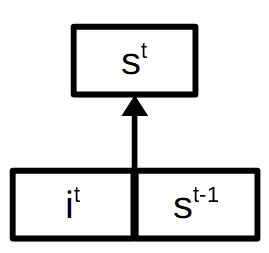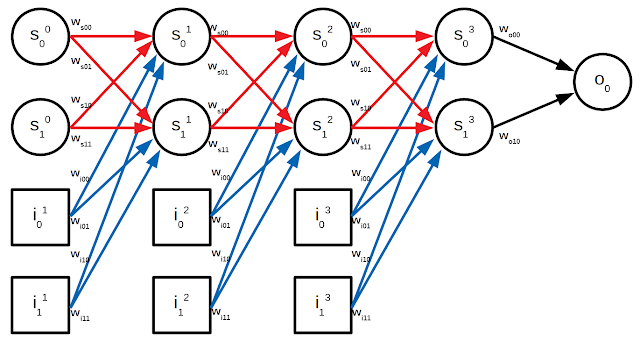## Wednesday, November 29, 2017

### An introduction to recurrent neural networks: Simple RNNs

Traditional feed forward neural networks work by taking a single fixed-length vector of inputs and producing a single fixed-length vector of outputs. After being trained on a training set, the neural network should be able to not only map inputs in the training set to their correct outputs, but also do so with new unseen inputs. The network is able to generalise to new inputs, but the new inputs must be of the same size. The network is not to generalise across complexity. For example if you train the network to perform addition on 4 digit numbers, it will not be able to perform addition on 5 digit numbers or even 3 digit numbers. Likewise if it learns to understand 5 word sentences then it will not be able to do anything with 6 word sentences. With images we usually solve this by resizing differently sized images into a standard size. This is not as straight forward to do with things like sentences. This is where recurrent neural networks come in.

Recurrent neural networks (RNNs) are used to give a neural network a short term memory which is used to be able to read a sequence of inputs and remember just a summary of what is important in the sequence. This summary, which would be a fixed-length vector called a state, can then be used by the rest of the neural network as usual. It can be used to predict the next word in a partial sentence or to determine the sentiment of a sentence. A simple RNN is a feed forward neural network where neurons in a layer have extra connections that loop around to the same layer as shown below:The figure shows a neural network consisting of 2 inputs and a state of neurons. The red connections allow each state neuron to produce an output based on a combination of the input neurons and the state neurons themselves. In other words the next state vector is produced based on a combination of the current input and previous state. This is the basis of short-term memory and the result is that after being exposed to a number of inputs, the state will be a vector that is influenced by each of the input vectors. The point is to train the neural network to remember what is important according to the task at hand. This means that we also need to use the final state (after processing all inputs) to generate an output (the state itself is not usually a useful output) which will make the RNN learn a useful representation of the input sequence.How are the inputs and the recurrent connections combined together? By concatenating the input and state vectors together and then passing them through a weight matrix as usual, generating the next state vector.But what happens for the first input? What's the first input going to concatenated with if there is no previous state? We have to define a default initial state for the first input. This is usually the all zeros vector but you can instead learn a constant vector that gets optimized during training.

Great, so that's all the basics sorted. Now for the formal notation. It is common to use the terminology of time series when talking about RNNs such that each input in a sequence belongs to a different time step in a series. In our formal notation, let's use superscripts to refer to time steps such that the first time step is 1 and the number of time steps is $T$.

$$s^0 = \mathbf{0}$$
$$s^t = f_s([s^{t-1} i^t] W_s + b_s)$$
$$o = f_o(s^T W_o + b_o)$$

where $s^t$ is the state vector at time $t$, $i^t$ is the input vector at time $t$, $o$ is the output vector, $\mathbf{0}$ is the all zeros vector, $f_s$ and $f_o$ are the activation functions of the state vector and output vector respectively, $W_s$ $b_s$ $W_o$ and $b_o$ are the weights and biases of the state vector and output vector respectively.

The question is how to learn the parameters of a recurrent function, which is not as single as with a feed forward neural network. The first thing we need to do is to unroll the recurrent network into a linear network that reuses the same parameters throughout. Although an RNN can be unrolled to infinity it is also the case that you only need to unroll it as far as your input sequences require. So if your training set contains an input sequence that is 3 time steps long, then you can use an RNN that is unrolled 3 times like this:Now it makes more sense and we can view it as a feed forward neural network. In fact an RNN is a feed forward network with the constraint that corresponding weights across time steps have to be identical. Notice how $W_{s00}$ and $W_{i00}$ are repeated with every time step. So whereas the weights in different layers in a feed forward neural net can be different, in an RNN they have to be the same. You might be thinking about how to handle the other input sequences of different lengths in the training set. We'll get to that later. For now let's assume that all sequences in the training set are grouped by length and that each minibatch consists of same length sequences.

Since training will involve finding the gradient of the loss with respect to each weight, let's start by finding the gradient of the output with respect to a sample of weights. If you're not familiar with the back propagation algorithm and how gradients are used to train neural networks in general you should check out this previous blog post before continuing on.

$$\frac{do_0}{dW_{o00}} = \frac{d}{dW_{o00}}f_o(W_{o00}s_0^3 + W_{o10}s_1^3) = f_o'(\ldots)s_0^3$$

That was straight forward. What about for recurrent weights?

$$\frac{do_0}{dW_{s00}} = \frac{d}{dW_{s00}}f_o(W_{o00}s_0^3 + W_{o10}s_1^3)$$
$$= f_o'(\ldots)(\frac{d}{dW_{s00}}f_s(W_{s00}s_0^2 + W_{s10}s_1^2 + W_{i00}i_0^3 + W_{i10}i_1^3) + \frac{d}{dW_{s00}}f_s(W_{s01}s_0^2 + W_{s11}s_1^2 + W_{i01}i_0^3 + W_{i11}i_1^3))$$
$$= f_o'(\ldots)(f_s'(\ldots)(\frac{d}{dW_{s00}}W_{s00}s_0^2))$$

And it is at this point that we will realise that things are more complicated than usual with feed forward neural nets. This is because $s_0^2$ can be decomposed to reveal more terms that contain $W_{s00}$ which means that we need to use the product rule.

$$= f_o'(\ldots)(f_s'(\ldots)(s_0^2\frac{d}{dW_{s00}}W_{s00} + W_{s00}\frac{d}{dW_{s00}}s_0^2))$$
$$= f_o'(\ldots)(f_s'(\ldots)(s_0^2 + W_{s00}\frac{d}{dW_{s00}}f_s(W_{s00}s_0^1 + W_{s10}s_1^1 + W_{i00}i_0^2 + W_{i10}i_1^2)))$$

...and so on, which would require as many decompositions as the length of the sequence. This is not compatible with the back propagation algorithm as it's not easy to extract a pattern that works for any sequence length. Keep in mind that we need to do this for the input weights as well.

Fortunately there is a simple solution: Treat all weights as being different and then add together the corresponding derivatives. What this means is that you put a superscript on each weight which indicates the time step it belongs to, hence making each weight different. So instead of having $W_{s00}$ we'd have $W_{s00}^3$, $W_{s00}^2$ and $W_{s00}^1$. Then we find the derivatives of each separate weight and finally add them all up:

$$\frac{do_0}{dW_{s00}} = \frac{do_0}{dW_{s00}^3} + \frac{do_0}{dW_{s00}^2} + \frac{do_0}{dW_{s00}^1}$$

This allows us to use normal back propagation to find each individual weight as if we were working on a feed forward neural net and then finally just add together corresponding derivatives in order to keep the weights identical. Notice that this is not a hack to force the weights to remain identical. The sum of the subderivatives really does equal $\frac{do_0}{dW_{s00}}$. You can try proving it yourself by trying to find the derivative using product rules as I was doing before. This trick is called back propagation through time.

We now get back to the question of handling variable length sequences in a training set. The solution to this is to make all sequences of equal length by padding them with pad vectors (the all zeros vector for example) and then make the RNN simply return the current state unmodified if the input is a pad vector. That way the state will remain the same beyond the length of the sequence, as if there were no pad vectors. This is the new RNN equation:

$$s^0 = \mathbf{0}$$
$$s^t = \begin{cases} f_s([s^{t-1} i^t] W_s + b_s) & \quad \text{if } i^t \text{ is not a pad}\\ s^{t-1} & \quad \text{otherwise} \end{cases}$$
$$o = f_o(s^T W_o + b_o)$$

You can now see how to implement a language model which predicts the next word in a partial sentence using Tensorflow by checking this previous blog post. You might also want to learn about how to represent words as vectors using word embeddings in this other blog post.